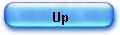CORDIC for dummies

The CORDIC algorithm is still mysterious to many. Here is a presentation using only simple arithmetic.

In the late 50’s Convair designed for the USAF, the B-58 Hustler supersonic jet bomber.

In 56, a project was started to replace the analog computer driven navigation system with a digital computer.

Jack Volder, at that time, senior engineer in the Convair’s aero electronics department discovered an algorithm to compute transcendental functions needed for real-time navigation problems.

He started is research by the « massaging of the basic angle addition equations » that he found in his « 1946 edition of the Handbook of Chemistry and Physics » :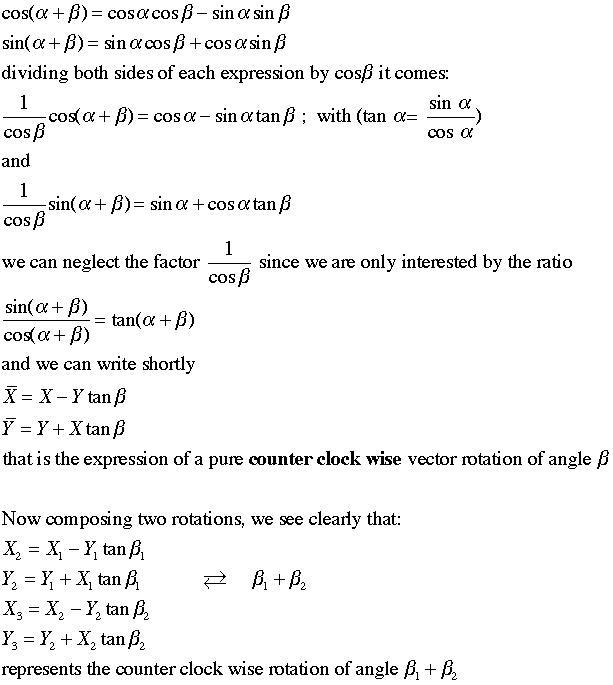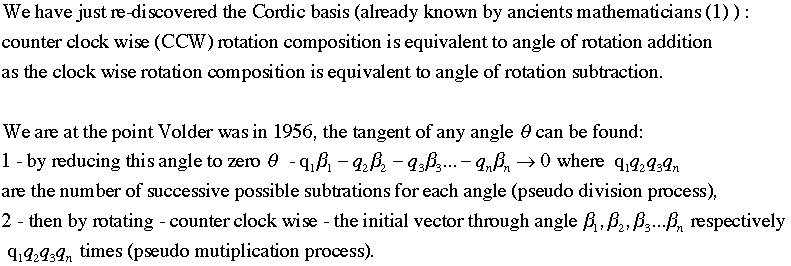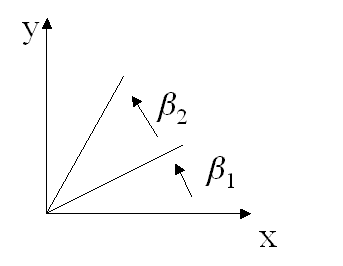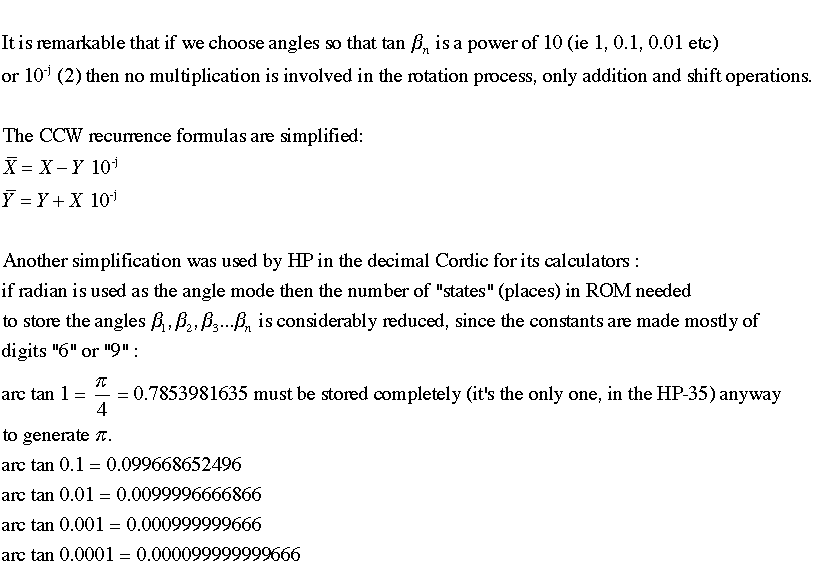Let’s take now a very simple example. The angle is 0.984736 radians and we are looking for the tangent.

We proceed by successive subtractions until overdraft. We can subtract only one time “arc tan” 1 and two times “arc tan” 0.1 :

 0.984736 0.785398163500000 1 -0.785398163500000 0.199337836500000 -0.586060327000000 KO -0.785398163500000 0.199337836500000 1 0.099669184004000 2 -0.099668652496000 0.000000531508000 -0.099668652496000 -0.099668120988000 KO -0.099668652496000

Now we start back with the residual vector (X = 1, Y = 0.000000531508000) and we rotate counter clock wise (CCW) two times through angle “arc tan” 0.1 and one time through angle “arc tan” 1 :

 CCW rotations X' Y' X Y X' = X - Y  10-j Y' = Y + X 10-j 1 0.999999947 0.100000532 1 0.000000531508000 2 0.989999894 0.200000526 0.999999947 0.100000531508000 1 0.789999368 1.19000042 0.989999894 0.200000526 Y/X= 1.50633085144

Important: there is no multiplication in the process, only shift right and subtraction operations

Look at the first CCW rotation, to calculate X’, two shift right (10-2 ) on Y   and a subtraction are enough (the « digits » are shifted right in this implementation ; but in the Volder’s original Cordic the bits are shifted).

 X 1,000000000000000 Y 0,000000531508000 0,000000005315080 (2 SR since 10-2) X’ = 0,999999994684920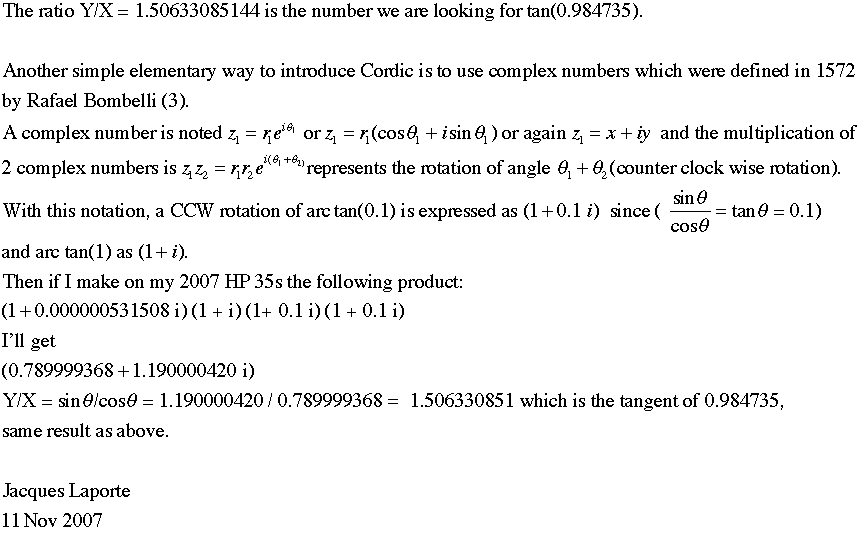_______________________________________________________________

(1) The addition formula is given by Abul Wafa in the 10th century:

(2) Implementing Cordic, Volder worked with powers of 2, because of the binary ALU. HP introduced the first decimal Cordic in the HP 9100 (03/1968).

(3)

NB: The underlying structure explaining that Cordic can be presented in terms of rotations or complex number is the orthogonal group SO(2,R).

See also for a unified “digit by digit” presentation of Volder’s Cordic and Meggitt’s pseudo division and pseudo multiplication method (and the links to download Volder’s and Meggitt’s work):